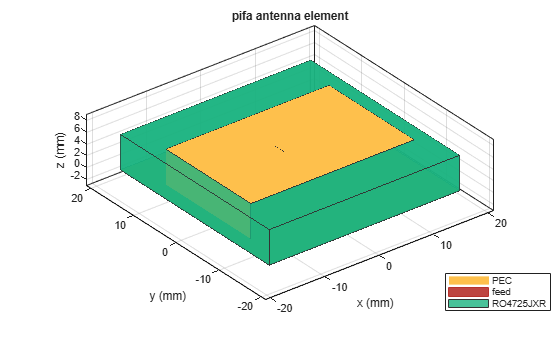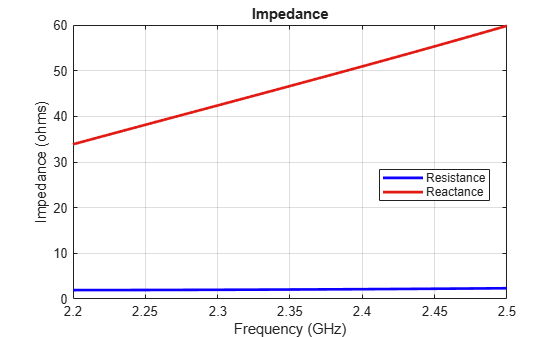# pifa

Create planar inverted-F antenna

## Description

The `pifa` object is a planar inverted-F antenna. The default PIFA antenna is centered at the origin. The feed point is along the length of the antenna.## Creation

### Syntax

``pf = pifa``
``pf = pifa(Name,Value)``

### Description

example

``` `pf = pifa` class to create a planar inverted-F antenna. ```

example

``` `pf = pifa(Name,Value)` class to create a planar inverted-F antenna, with additional properties specified by one, or more name-value pair arguments. `Name` is the property name and `Value` is the corresponding value. You can specify several name-value pair arguments in any order as `Name1`, `Value1`, `...`, `NameN`, `ValueN`. Properties not specified retain their default values.```

## Properties

expand all

PIFA antenna length, specified as a scalar in meters. By default, the length is measured along the x-axis.

Example: `'Length',75e-3`

Data Types: `double`

PIFA antenna width, specified as a scalar in meters. By default, the width is measured along the y-axis.

Example: `'Width',35e-3`

Data Types: `double`

Height of the substrate, specified as a scalar in meters.

Example: `'Height',37e-3`

Data Types: `double`

Type of dielectric material used as a substrate, specified as an object. For more information see, `dielectric`. For more information on dielectric substrate meshing, see Meshing.

Note

The substrate dimensions must be equal to the groundplane dimensions.

Example: ```d = dielectric('FR4'); 'Substrate',d```

Example: ```d = dielectric('FR4'); pf.Substrate = d```

Ground plane length, specified as a scalar in meters. By default, ground plane length is measured along the x-axis. Setting `'GroundPlaneLength'` to `Inf`, uses the infinite ground plane technique for antenna analysis.

Example: `'GroundPlaneLength',3`

Data Types: `double`

Ground plane width, specified as a scalar in meters. By default, ground plane width is measured along the y-axis. Setting `'GroundPlaneWidth'` to `Inf`, uses the infinite ground plane technique for antenna analysis.

Example: `'GroundPlaneWidth',2.5`

Data Types: `double`

Signed distance from the center along length and width of the ground plane, specified as a two-element vector in meters. Use this property to adjust the location of the patch relative to the ground plane.

Example: `'PatchCenterOffset',[0.01 0.01]`

Data Types: `double`

Shorting pin width of patch, specified as a scalar in meters. By default, the shorting pin width is measured along the y-axis.

Example: `'ShortPinWidth',3`

Data Types: `double`

Signed distance from center along length and width of ground plane, specified as a two-element vector. Use this property to adjust the location of the feedpoint relative to ground plane and patch.

Example: `'FeedOffset',[0.01 0.01]`

Data Types: `double`

Type of the metal used as a conductor, specified as a metal material object. You can choose any metal from the `MetalCatalog` or specify a metal of your choice. For more information, see `metal`. For more information on metal conductor meshing, see Meshing.

Example: ```m = metal('Copper'); 'Conductor',m```

Example: ```m = metal('Copper'); ant.Conductor = m```

Lumped elements added to the antenna feed, specified as a lumped element object. For more information, see `lumpedElement`.

Example: `'Load',lumpedelement`. `lumpedelement` is the object for the load created using `lumpedElement`.

Example: ```pf.Load = lumpedElement('Impedance',75)```

Tilt angle of the antenna, specified as a scalar or vector with each element unit in degrees. For more information, see Rotate Antennas and Arrays.

Example: `Tilt=90`

Example: `Tilt=[90 90]`,`TiltAxis=[0 1 0;0 1 1]` tilts the antenna at 90 degrees about the two axes defined by the vectors.

Note

The `wireStack` antenna object only accepts the dot method to change its properties.

Data Types: `double`

Tilt axis of the antenna, specified as:

• Three-element vector of Cartesian coordinates in meters. In this case, each coordinate in the vector starts at the origin and lies along the specified points on the X-, Y-, and Z-axes.

• Two points in space, each specified as three-element vectors of Cartesian coordinates. In this case, the antenna rotates around the line joining the two points in space.

• A string input describing simple rotations around one of the principal axes, 'X', 'Y', or 'Z'.

Example: `TiltAxis=[0 1 0]`

Example: `TiltAxis=[0 0 0;0 1 0]`

Example: `TiltAxis = 'Z'`

Data Types: `double`

## Object Functions

 `show` Display antenna, array structures or shapes `info` Display information about antenna or array `axialRatio` Axial ratio of antenna `beamwidth` Beamwidth of antenna `charge` Charge distribution on antenna or array surface `current` Current distribution on antenna or array surface `design` Design prototype antenna or arrays for resonance around specified frequency `efficiency` Radiation efficiency of antenna `EHfields` Electric and magnetic fields of antennas; Embedded electric and magnetic fields of antenna element in arrays `impedance` Input impedance of antenna; scan impedance of array `mesh` Mesh properties of metal, dielectric antenna, or array structure `meshconfig` Change mesh mode of antenna structure `optimize` Optimize antenna or array using SADEA optimizer `pattern` Radiation pattern and phase of antenna or array; Embedded pattern of antenna element in array `patternAzimuth` Azimuth pattern of antenna or array `patternElevation` Elevation pattern of antenna or array `rcs` Calculate and plot radar cross section (RCS) of platform, antenna, or array `returnLoss` Return loss of antenna; scan return loss of array `sparameters` Calculate S-parameter for antenna and antenna array objects `vswr` Voltage standing wave ratio of antenna

## Examples

collapse all

Create and view a PIFA antenna with 30 mm length, 20 mm width over a 35 mm x 35 mm ground plane, and feedpoint at (-2 mm,0,0).

`pf = pifa`
```pf = pifa with properties: Length: 0.0300 Width: 0.0200 Height: 0.0100 Substrate: [1x1 dielectric] GroundPlaneLength: 0.0360 GroundPlaneWidth: 0.0360 PatchCenterOffset: [0 0] ShortPinWidth: 0.0200 FeedOffset: [-0.0020 0] Conductor: [1x1 metal] Tilt: 0 TiltAxis: [1 0 0] Load: [1x1 lumpedElement] ```
`show(pf)`Plot the radiation pattern of a PIFA antenna at a frequency of 2.3 GHz.

```pf = pifa('Length',30e-3, 'Width',20e-3, 'GroundPlaneLength',35e-3,... 'GroundPlaneWidth',35e-3)```
```pf = pifa with properties: Length: 0.0300 Width: 0.0200 Height: 0.0100 Substrate: [1x1 dielectric] GroundPlaneLength: 0.0350 GroundPlaneWidth: 0.0350 PatchCenterOffset: [0 0] ShortPinWidth: 0.0200 FeedOffset: [-0.0020 0] Conductor: [1x1 metal] Tilt: 0 TiltAxis: [1 0 0] Load: [1x1 lumpedElement] ```
`pattern(pf,2.3e9);`Create a PIFA antenna using a dielectric substrate 'RO4725JXR'.

```d = dielectric('RO4725JXR'); pf = pifa('Length',30e-3, 'Width',20e-3,'Height',0.0060, 'GroundPlaneLength',35e-3, ... 'GroundPlaneWidth', 35e-3,'Substrate',d) show(pf) ```
```pf = pifa with properties: Length: 0.0300 Width: 0.0200 Height: 0.0060 Substrate: [1×1 dielectric] GroundPlaneLength: 0.0350 GroundPlaneWidth: 0.0350 PatchCenterOffset: [0 0] ShortPinWidth: 0.0200 FeedOffset: [-0.0020 0] Tilt: 0 TiltAxis: [1 0 0] Load: [1×1 lumpedElement] ```Calculate the impedance of the antenna over the specified frequency range. GHz.

```impedance(pf,linspace(2.2e9,2.5e9,31)); ```Balanis, C.A. Antenna Theory. Analysis and Design, 3rd Ed. New York: Wiley, 2005.